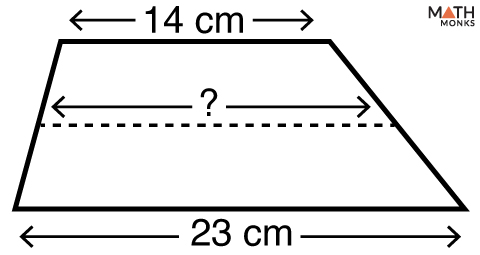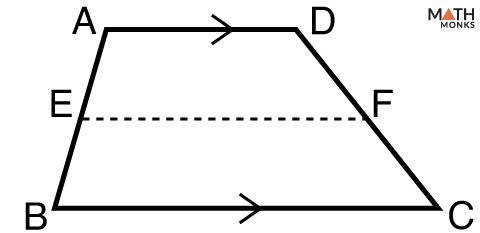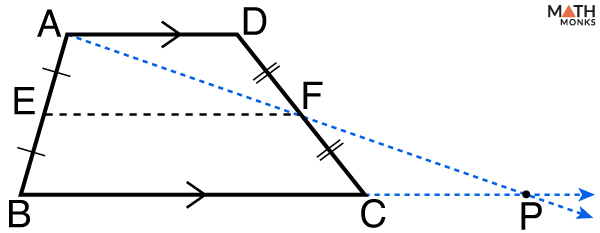# Midsegment (Median) of a Trapezoid

The mid-segment of a trapezoid is the line segment mid-way between its two bases.  It is also called the median or the midline.

How many mid-segments does a trapezoid have?

A trapezoid has only one mid-segment since it has only 2 parallel sides and 2 non parallel sides.

The mid-segment bisects the non parallel sides. In other words, a mid-segment is the mid-way between the two bases.

The next segment will help you find the mid-segment of a trapezoid.

## Formula

The basic formula to find the mid-segment of a trapezoid is given below:Find the length of the mid-segment of the trapezoid given.

Solution:

As we know,
Mid-segment (M) = ½(a + b), here a = 23 cm, b = 14 cm
∴ M = ½(23 + 14)
= 18.5 cmIn trapezoid PQRS, what is the length of mid-segment XY.

Solution:

As we know,
Mid-segment (M) = ½(27 + 12), here a = 27 cm, b = 12 cm
∴ M = ½(27 + 12)
= 19.5 cm

## Trapezoid Midsegment Theorem

The trapezoid mid-segment theorem states that a line connecting the midpoints of the non-parallel sides (legs) is parallel to the bases. It measures half the sum of lengths of the bases.

### Trapezoid Midsegment Theorem ProofProve that the line connecting the midpoints of the legs of a trapezoid ABCD is parallel to the bases and half the sum of lengths of the bases.

To prove: